# EQUATION

Specifies the equation systems present in the problem.

AcuTrace Command

## Syntax

EQUATION {parameters}

## Qualifier

This command has no qualifier.

## Parameters

particle (enumerated) [=massless]
Type of the particle motion equation.
massless
Massless particle equation.
finite_mass
Finite mass particle equation.
stretch (enumerated) [=none]
Type of the stretch equation.
none
No stretch equation present.
standard
Standard stretch equation.
user_equations (list) [={}]
List of user equations to be used.
number_particle_components or num_part_comps (integer) >=0 [=0]
Number of particle components.

## Description

The EQUATION command specifies the existence and types of the equation systems and solution fields present in the problem. For example, to specify a problem with particle position using the massless model and stretch, you need:
EQUATION {
particle = massless
stretch  = standard
}
while to use the finite mass model instead, you need:
EQUATION {
particle = finite_mass
stretch  = standard
}

In the massless model, the velocity of a particle always equals the flow velocity at the particle's location. In the finite mass model, the particle velocity does not necessarily equal the flow velocity. Instead, the particles have mass and are subject to forces such as drag and gravity.

The massless particle and stretch equations are:(1)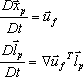where $\frac{D}{Dt}$ denotes the derivative in the particle frame of reference, t is time, ${\stackrel{\to }{x}}_{p}$ is the particle position, ${\stackrel{\to }{u}}_{f}$ is the flow velocity, $\nabla {\stackrel{⇀}{u}}_{f}{}^{T}$ is the transpose of the velocity gradient, and ${\stackrel{⇀}{l}}_{p}$ is the stretch vector.

If the parameter turbulence_trace in the TRACE_PARAMETERS command is set to on, the massless particle equation solved is not the above but instead(2)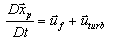where ${\stackrel{⇀}{u}}_{turb}$ is a random perturbation to the particle velocity based on the eddy viscosity.

The finite mass particle equations are(3)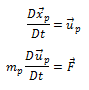where ${\stackrel{\to }{u}}_{p}$ is the particle velocity, ${m}_{p}$ is the particle mass, and $\stackrel{\to }{F}$ is the sum of the forces acting on the particle. If the parameter turbulence_trace in the TRACE_PARAMETERS command is set to on, then the values of ${\stackrel{\to }{u}}_{f}$ used in evaluating $\stackrel{\to }{F}$ are replaced by ${\stackrel{\to }{u}}_{f}+{\stackrel{\to }{u}}_{turb}$ .
Note: Particles are assumed to be spherical of constant (subgrid scale) size.
The particle and stretch equations can be augmented by one or more user equations. The user equations can either be evolution equations of the form(4)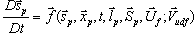where ${\stackrel{⇀}{s}}_{p}$ is the set of user-defined particle variables in the current equation, ${\stackrel{⇀}{S}}_{p}$ is the set of user-defined variables from the other user equations (if any), ${\stackrel{⇀}{U}}_{f}$ is the set of flow variables, and ${\stackrel{⇀}{V}}_{udf}$ is the set of user equation parameters; or evaluation equations of the form(5)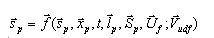The user equations present in the problem are specified by the user_equations parameter. Each user equation specified by the user_equations parameter must be defined by a USER_EQUATION command.

For example, evolution equations tparticle and cparticle for particle temperature and composition could be defined. To include these equations in a problem, one could have:
EQUATION {
...
user_equations = {tparticle, cparticle}
}
USER_EQUATION( "tparticle" ) {
user_function = "usrTparticle"
num_variables = 1
...
}
USER_EQUATION( "cparticle" ) {
user_function = "usrCparticle"
num_variables = 2                         # 2 species example
...
}
The EQUATION command specifies the existence and types of the equation systems and solution fields present in the problem. The TIME_SEQUENCE and STAGGER commands must then be used to request the solution for the specified equations. For example, to solve for particle position and stretch, you could have:
EQUATION {
particle = massless
stretch = standard
}
TIME_SEQUENCE {
staggers = { "particle", "stretch" }
...
}
STAGGER( "particle" ) {
equation = particle
...
}
STAGGER( "stretch" ) {
equation = stretch
...
}
As with particle and stretch equations, the TIME_SEQUENCE and STAGGER commands must be used to request the solution for the specified user equations. For example, to solve for particle position, temperature, and composition, you could have:
EQUATION {
particle       = massless
user_equations = {tparticle, cparticle}
}
TIME_SEQUENCE {
staggers       = { "particle", "tparticle", "cparticle"}
...
}
STAGGER( "particle" ) {
equation = particle
...
}
STAGGER( "tparticle" ) {
equation      = user_equation
user_equation = "cparticle"
...
}
STAGGER( "cparticle" ) {
equation      = user_equation
user_equation = "tparticle"
...
}
USER_EQUATION( "tparticle" ) {
user_function = "usrTparticle"
num_variables = 1
...
}
USER_EQUATION( "cparticle" ) {
user_function = "usrCparticle"
num_variables = 2
...
}

If an equation is defined by the EQUATION command, but no active stagger references that equation, the solution field of that equation is unaltered throughout the analysis.

Particle components are a generalization of a marker variable; they can also be considered a generalization of species or composition. When number_particle_components is greater than zero, particle components are initialized in the PARTICLE_SEED command. The values of the particle components are constant in time but they may vary over the particles in the problem according to how they are initialized.